### ELECTRODYNAMIC AND ELECTROMAGNETIC ACTIONS

WALTER RITZ

#### Annales 233 (Oeuvres 390)

Letbe the positive and negative charges of two elements in two wires, one situated at x', y', z', the other at x, y, z. By hypothesis, the total charges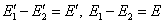are very small in relation to,… The force exerted by ds' on ds is the sum of actions ofand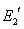onand. The speeds of the positive ions and of the substance are V, V'; those of the negative ions, v, v'; the relative speeds V-v=η, V'-v'= η', and consequently, J, J' have the direction (Oeuvres 391)ds (dx, dy, dz) and ds' (dx', dy', dz'); thus we will have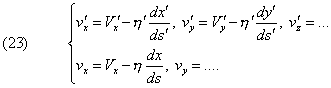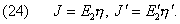Let us put these values in expression (13) of Fx, and do the indicated sum. The term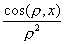will have a coefficient of;

this is the electrostatic action.

The terms in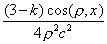will be, to a factor of about ds ds',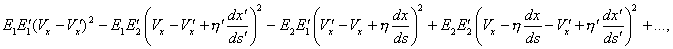the non-written terms being deduced from these by circular permutation of x y z. The expression may be written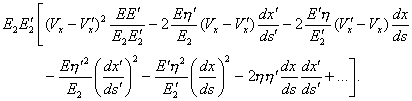When the resulting charges E, E' are nil, only the last one subsist. In the other cases,are very small, and as the whole must be multiplied by 1/c2, one can see that the first term is absolutely negligible compared to the electrostatic term. The second term corresponds to a very weak action of a neutral current on an electrostatic charge in motion, the third one to the action of such a charge on a current or a magnet (Rowland effect). These two effects are of the same order of magnitude, and manifest themselves only (Oeuvres 392) in very delicate experiments and when V or V' are considerable. If the electrostatic term itself is weak, as it is in general, they are absolutely negligible. We will discuss the Rowland effect later on.

The squared terms are also very small in relation to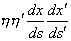, and only this last term remains:Likewise, the terms inand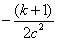perceptibly give only a result proportional to JJ', and we will have, for the ensemble of terms dependent on

the speeds,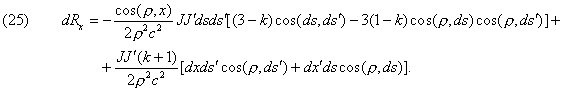According to Lorentz’s formula (20), the action of ds' on ds is, by virtue of analogous reasoning, given by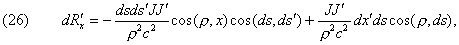the terms in E, E' and the squared terms again being negligible. As for the term containing the accelerations, which is the same in both theories, it is again multiplied by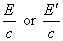, and is consequently negligible, unless the accelerations are very large, which isn’t the case under experimental conditions where one can observe the electrodynamic or electromagnetic actions.

I say that the resultant of the action of a closed circuit ds' on the element ds is the same following (25) and (26). In fact,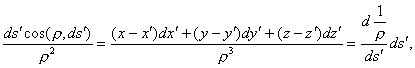(Oeuvres 393) because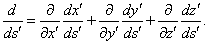The term in dx ds' cos(ρ,ds') is consequently a total differential in relation to s'; its integral is nil for

a closed contour. Likewise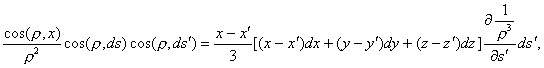and the integration by parts along s' will transform this term into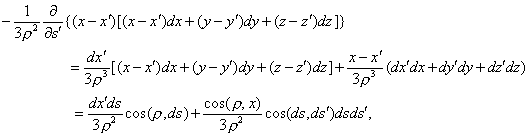so that it comes, for the sought resulting action experienced by ds,This is exactly what the integration of (26) would give. The constant k disappears from the result. Besides, the latter is independent of the motion of the wires or of their deformation, provided that J and J' stay constant, that the element of current J ds be neutral, and that J' be closed and neutral. The rotational actions of the currents and the magnets are thus explained in a classical manner.

For k= -1, the action of two elements of neutral current would be exactly given by the formula of Ampère; this formula may thus be admitted even today.

When there is a question about a body of two or three dimensions, of which one is traversed by a neutral current decomposable into closed filaments, the latter acts on the other elements of volume in accordance to the formula obtained; but, furthermore, the currents themselves slightly change their direction, as we shall see in the following (Oeuvres 394) section: this is the Hall effect. But this effect is minimal, and may be disregarded here.

As always, the effects of magnetism will be obtained by replacing magnets by molecular closed neutral currents; here again, there will thus be no difference between the theories.